# VLOOKUP with Multiple Criteria in Google Sheets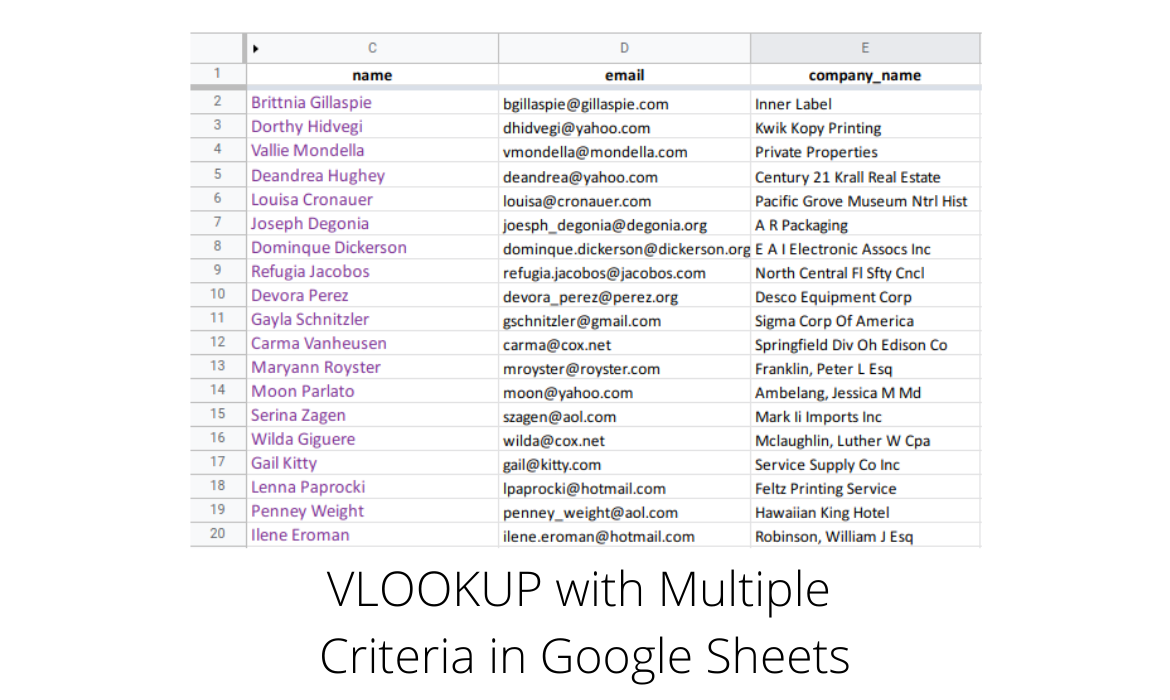One of the more common tasks in any spreadsheet application is searching through the data.

Google sheets already comes with a very neat and powerful find option in the toolbar which searches for a keyword anywhere in the sheet.

But there are times however, when you want to do something a bit more complex. Perhaps it’s a search that takes into account two or more columns at the same time.

Or you want the search to return a piece of information related to the search term, such as looking up a name and receiving the corresponding email address.

Oftentimes, you’ll also want to output the search results into a cell, or use them in a formula.

To do all these, we’re going to be looking at a few handy Google sheets functions and using the following example sheet, which is a very simple mailing list.We want to be able to lookup a person’s name and have the sheet return the email address or company associated with that person.

Ideally, we’d like it so that if we change the name to search for, the result also updates, automatically.

As it turns out, Google sheets has a function called VLOOKUP (which stands for Vertical LOOKUP), that does exactly this.

The following is taken from the Google Docs Editors Help definition for the function.

VLOOKUP

Vertical lookup. Searches down the first column of a range for a key and returns the value of a specified cell in the row found.

### Sample Usage

VLOOKUP(10003, A2:B26, 2, FALSE)

### Syntax

VLOOKUP(search_key, range, index, [is_sorted])

• search_key – The value to search for. For example, 42, “Cats”, or I24.
• range – The range to consider for the search. The first column in the range is searched for the key specified in search_key.
• index – The column index of the value to be returned, where the first column in range is numbered 1.
• If index is not between 1 and the number of columns in range, #VALUE! is returned.
• is_sorted – [TRUE by default] – Indicates whether the column to be searched (the first column of the specified range) is sorted. FALSE is recommended in most cases.
• It’s recommended to set is_sorted to FALSE. If set to FALSE, an exact match is returned. If there are multiple matching values, the content of the cell corresponding to the first value found is returned, and #N/A is returned if no such value is found.
• If is_sorted is TRUE or omitted, the nearest match (less than or equal to the search key) is returned. If all values in the search column are greater than the search key, #N/A is returned.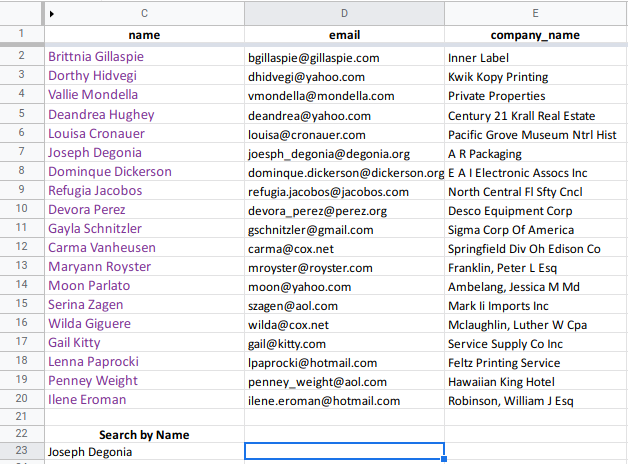We’ll begin with a simple example so that we can become familiar with how VLOOKUP works.

At the bottom of our sheet, we’ve assigned cell C23 as the field where we can enter a name, and we want to have the company name printed in the the adjacent cell, D23.The formula required to do this is:
=VLOOKUP(C23, C2:E20, 3, 0)

Looking at the syntax for VLOOKUP, we see that the first parameter (which references cell C23), is the search key or value we are searching for.

In our example, we’ve entered Joseph Degonia. This name appears in the seventh row of our mailing list, so we expect the formula to find a match.

Next comes the range of cells encompassed by the search, C2:E20.

The range can span across several columns and down any number of rows.

The only restriction is that only the first column in the range is used in the search for the search key provided in the first parameter.

In other words, our range starts from cell C2 and ends at cell E20, which would make column C the first column in the range.

VLOOKUP will thus only look in column C to find a match against our search key (the name we enter in cell C23), which at the moment contains Joseph Degonia.

The third parameter, which in our formula has a value of 3, tells VLOOKUP from which column to pull the results.

Since our range spans from column C to column E (C2:E20), column C is considered the first column in the range, and therefore the 3rd column would be column E.

The last parameter simply tells VLOOKUP whether the column on which the search is carried out is sorted or not.

The resulting company name returned (in cell D23) is A R Packaging.

Since the search on column C would have found a match in row 7 in the sheet, it then crosses over to the 3rd column in the range (column E) in the same row, and pulls out the result.

That was a fairly straightforward search.

Our next search is going to be a little bit more difficult, but not by much.

The name to search for will be held in two cells, one holding the first name, the other the last name.

This may not seem very practical in this particular situation, but as an exercise it does highlight how to build a search key that is a composite of more than one cell.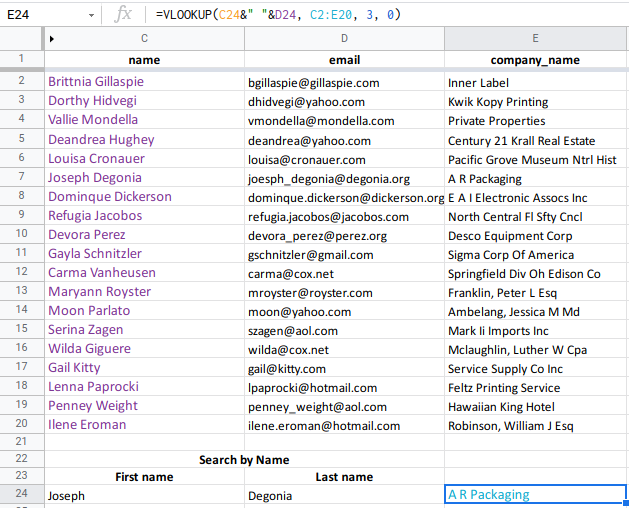The formula now becomes:
=VLOOKUP(C24 & ” ” & D24, C2:E20, 3, 0)

The only change is in the way we reference the search key or name, which is now a merger of cells C24 and D24.

Cell C24 contains the first name, and D24 the last name.

The first parameter to VLOOKUP changes from the single C23, to what is known as a concatenation of the cells C24 and D24, C24&” “&D24.

The ampersand (&) is the concatenation operator and simply means add together the strings before and after the operator.

In this case, to the contents of C24 is being appended the space character (enclosed in the double quotes “ “), to which in turn are being appended the contents of D24.

We need to include a space, otherwise the resulting concatenation would produce JosephDegonia, and would never find a match, regardless of what names we entered.

We now move on to a slightly more complex situation, in which the names in our mailing list are not in the one column, but are in two separate columns, first_name and last_name.Adapting the formula from before (to account for the change in column lettering after splitting the name into two columns), produces the error, Did not find value ‘Joseph Degonia’ in VLOOKUP evaluation.

If you remember from earlier, VLOOKUP uses the first and only the first column defined in the range to do its search.

But now, the first column only contains the first name. So how do we tell VLOOKUP to search through the merger of columns A and B?

Not surprisingly, there is a function to do that as well, called ARRAYFORMULA.

Again from Google Docs Editors Help, the definition for ARRAYFORMULA:

ARRAYFORMULA

Enables the display of values returned from an array formula into multiple rows and/or columns and the use of non-array functions with arrays.

### Sample Usage

ARRAYFORMULA(SUM(IF(A1:A10>5, A1:A10, 0)))

ARRAYFORMULA(A1:C1+A2:C2)

### Syntax

ARRAYFORMULA(array_formula)

• array_formula – A range, mathematical expression using one cell range or multiple ranges of the same size, or a function that returns a result greater than one cell.

Let’s play around with ARRAYFORMULA to see just what it can do. We’ll proceed one step at a time.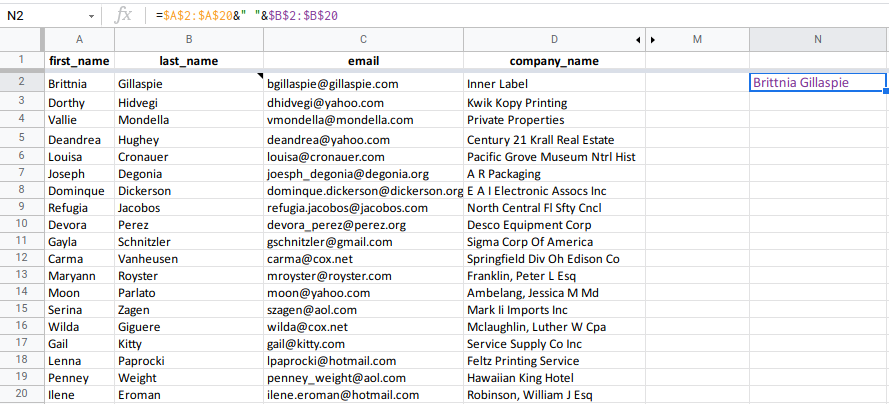Here is our contact list and we’ve started by entering our first attempt at a formula in cell N2, that tries to merge the first and last name columns:

=\$A\$2:\$A\$20 & ” ” & \$B\$2:\$B\$20

It almost works, but it only merges a single name, whereas we want it to do this for the entire list of names.

If we can achieve this, we can then use it as the VLOOKUP function’s second parameter.

The problem lies in the fact that the formula returns a single name whereas we want the full range of names.

The function ARRAYFORMULA comes to the rescue, and is capable of returning an array (or range) of values.

What is called a range in a spreadsheet, is more commonly referred to as an array in programming, hence the function name.If we now wrap our formula inside the ARRAYFORMULA function, =ARRAYFORMULA(\$A\$2:\$A\$20 & ” ” & \$B\$2:\$B\$20) we get the result shown in column N in the above screenshot.

It has merged the first and last names (columns A and B) into a single array and displayed them in column N.

While this is a step in the right direction, we are still not there. The range we pass to VLOOKUP also has to include columns C and D.

So to the above formula, we need to also add those two columns.

Since ARRAYFOMULA only takes one parameter, we need to use brackets (or curly braces) {}.

Brackets allow you to group together values. When the values are separated by a comma, they are placed in adjacent columns.

For example,  ={1, 2} would place the number 1 in the current cell and the number 2 in the cell to the right in the adjacent column.

Applying this to our formula, we can now create a range that includes the merged first and last names, as well as the email and company_name columns.The formula to create the complete range now becomes:

=ARRAYFORMULA({\$A\$2:\$A\$20 & ” ” & \$B\$2:\$B\$20, \$C\$2:\$D\$20})

The brackets allow us to have our merged first and last names, as well as columns C and D, and the result is what we see in columns N – P in the snapshot above.

We can now plug this into VLOOKUP as the second parameter, which defines the range of the lookup.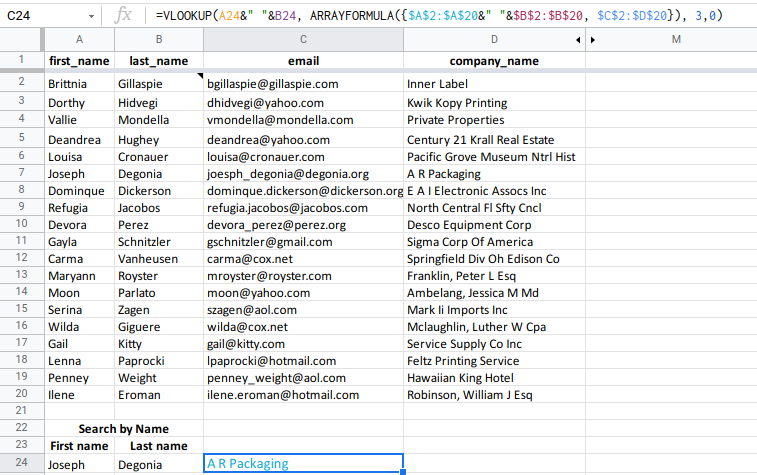The final and completed formula is as follows, and the result is shown in cell C24.

=VLOOKUP(A24&” “&B24, ARRAYFORMULA({\$A\$2:\$A\$20&” “&\$B\$2:\$B\$20, \$C\$2:\$D\$20}), 3,0)

As a bonus, I’m going to provide an alternative solution that uses the QUERY function.

If you are familiar with SQL queries, then Google sheet’s QUERY will be easy to grasp.

QUERY function

Runs a Google Visualization API Query Language query across data.

### Sample Usage

QUERY(A2:E6,”select avg(A) pivot B”)

QUERY(A2:E6,F2,FALSE)

### Syntax

• data – The range of cells to perform the query on.
• Each column of data can only hold boolean, numeric (including date/time types) or string values.
• In case of mixed data types in a single column, the majority data type determines the data type of the column for query purposes. Minority data types are considered null values.
• query – The query to perform, written in the Google Visualization API Query Language.
• headers – [ OPTIONAL ] – The number of header rows at the top of data. If omitted or set to -1, the value is guessed based on the content of data.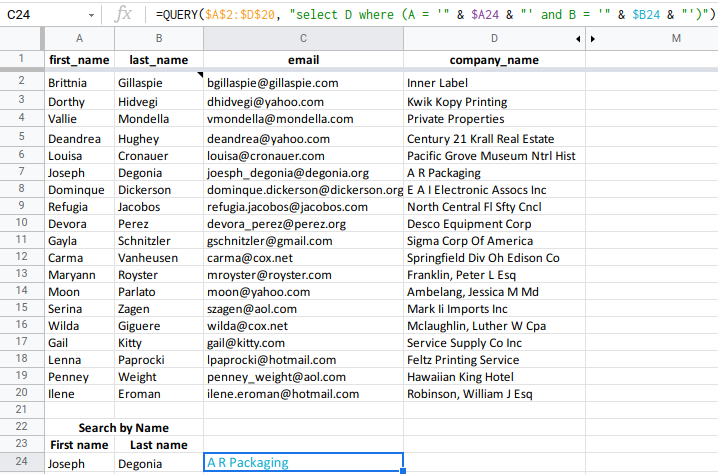The formula is as follows:

=QUERY(\$A\$2:\$D\$20, “SELECT D WHERE (A = ‘” & \$A24 & “‘ AND B = ‘” & \$B24 & “‘)”)

The first parameter defines the range of cells with the data, while the second parameter contains the query statement, which is where we carry out our search.

Let’s quickly analyze the query.

• SELECT D : If a match is found, then it selects the result along the matching row from column D.
• WHERE (….) : The where clause defines what conditions constitute a match. The first part of the clause checks to see if there’s a match in column A against the contents of cell A24. The second part checks to see if there’s a match in column B against the contents of cell B24. The AND signifies that both conditions must be met in order for a match to occur. The where clause equates to WHERE (A = ‘Joseph’ AND B = ‘Degonia’)

The QUERY function can be a very powerful tool, especially if you are familiar with the Structured Query Language (SQL), otherwise there is a bit of a learning curve, but definitely worth the effort.

If we compare the VLOOKUP formula with the QUERY formula, the QUERY formula is simpler, cleaner and more intuitive.

Costas has a wide range of experience in Information Technology covering computer hardware, programming, telecommunications, networking, web services, and general IT support. He's worked in various roles such as PHP programmer and web developer, technical and desktop support, hardware repair, system administration. Costas has excellent background in Microsoft Windows and Office Suite (Excel, Word, Powerpoint, etc), as well as a thorough understanding of Networking and Hardware maintenance.